Download Presentation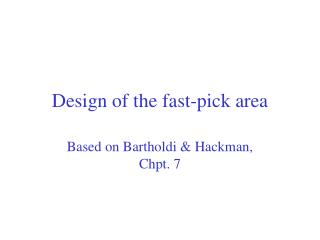Design of the fast-pick area

# Design of the fast-pick area - PowerPoint PPT Presentation

Design of the fast-pick area. Based on Bartholdi & Hackman, Chpt. 7. The “fast-pick” or “forward-pick” or “primary-pick” area. Primary picking. Restocking. Shipping. Receiving. Forward pick Area. Reserves picking. Reserves Area.I am the owner, or an agent authorized to act on behalf of the owner, of the copyrighted work described.
Download Presentation## Design of the fast-pick area

An Image/Link below is provided (as is) to download presentation

Download Policy: Content on the Website is provided to you AS IS for your information and personal use and may not be sold / licensed / shared on other websites without getting consent from its author.While downloading, if for some reason you are not able to download a presentation, the publisher may have deleted the file from their server.

- - - - - - - - - - - - - - - - - - - - - - - - - - E N D - - - - - - - - - - - - - - - - - - - - - - - - - -
Presentation Transcript### Design of the fast-pick area

Based on Bartholdi & Hackman, Chpt. 7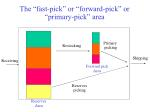The “fast-pick” or “forward-pick” or “primary-pick” area

Primary

picking

Restocking

Shipping

Receiving

Forward pick

Area

Reserves

picking

Reserves

Area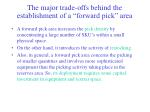The major trade-offs behind the establishment of a “forward pick” area
• A forward pick area increases the pick density by concentrating a large number of SKU’s within a small physical space.
• On the other hand, it introduces the activity of restocking.
• Also, in general, a forward pick area concerns the picking of smaller quantities and involves more sophisticated equipment than the picking activity taking place in the reserves area. So, its deployment requires some capital investment in equipment and (extra) space.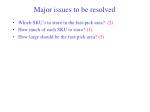Major issues to be resolved
• Which SKU’s to store in the fast-pick area? (2)
• How much of each SKU to store? (1)
• How large should be the fast-pick area? (3)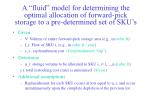A “fluid” model for determining the optimal allocation of forward-pick storage to a pre-determined set of SKU’s
• Given
• V: Volume of entire forward-pick storage area (e.g., in cubic ft)
• f_i: Flow of SKU i, (e.g., in cubic ft / year)
• c_r : replenishment cost (\$/replenishment “trip”)
• Determine
• u_i: storage volume to be allocated to SKU i, i=1,…,n (cubic ft)

s.t. total restocking cost (rate) is minimized (\$/year)

• Replenishment for each SKU occurs at lots equal to u_i, and occur instantaneously upon the complete depletion of the previous lot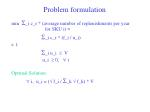Problem formulation

min _i c_r * (average number of replenishments per year for SKU i) =

_i c_r * (f_i / u_i)

s. t.

_i u_i  V

u_i  0,  i

Optimal Solution:

 i, u_i = ( f_i / _k  f_k) * V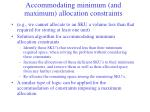Accommodating minimum (and maximum) allocation constraints
• (e.g., we cannot allocate to an SKU a volume less than that required for storing at least one unit)
• Solution algorithm for accommodating minimum allocation constraints
• Identify those SKU’s that received less than their minimum required space, when solving the problem without considering these constraints.
• Increase the allocations of these deficient SKU’s to their minimum requirements, and remove them as well as their allocated space from any further consideration.
• Re-allocate the remaining space among the remaining SKU’s.
• A similar type of logic can be applied for the accommodation of constraints imposing a maximum allocation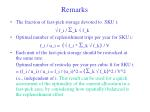Remarks
• The fraction of fast-pick storage devoted to SKU i:

 f_i / _k  f_k

• Optimal number of replenishment trips per year for SKU i:

f_i / u_i = ( f_i * _k  f_k) / V

• Each unit of the fast-pick storage should be restocked at the same rate:

Optimal number of restocks per year per cubic ft for SKU i = (f_i / u_i) / u_i = f_i / (u_i)^2 = (_k  f_k)^2 / V^2

i.e., independent of i. This result can be used for a quick assessment of the optimality of the current allocation in a fast-pick area, by considering how (spatially) balanced is the replenishment effort.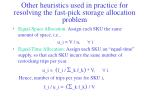Other heuristics used in practice for resolving the fast-pick storage allocation problem
• Equal-Space Allocation: Assign each SKU the same amount of space, i.e.,

u_i = V / n,  i

• Equal-Time Allocation: Assign each SKU an “equal-time” supply, so that each SKU incurs the same number of restocking trips per year.

u_i = (f_i / _k f_k) * V,  i

Hence, number of trips per year for SKU i,

f_i / u_i = (_k f_k) / V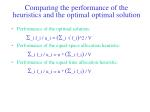Comparing the performance of the heuristics and the optimal optimal solution
• Performance of the optimal solution:

_i f_i / u_i = (_i  f_i)^2 / V

• Performance of the equal-space allocation heuristic:

_i f_i / u_i = n * (_i f_i) / V

• Performance of the equal-time allocation heuristic:

_i f_i / u_i = n * (_i f_i) / VA statistical assessment of the sub-optimality of the equal-space/time allocation

Perf. of heuristics / Perf. of optimal sol. =

_i f_i / n

(_i  f_i / n)^2

Assume that each  f_i is an independent sample from a random

variable Y with mean m and variance s^2. Then, the above ratio is approximated by:

m^2 + s^ 2

= 1 + CV^2

m^2

Hence, the more diverse the rates of flow of the various SKU’s,

the more sub-optimal is the performance of the two heuristics.• To resolve this issue, one must quantify the “net benefit” of having the SKU in the fast-pick area vs. doing all the picking from the reserve.
• This is done as follows: Let
• V: Volume of entire forward-pick storage area (e.g., in cubic ft)
• f_i: Flow of SKU i, (e.g., in cubic ft / year)
• c_r: cost of each restock trip (\$/trip)
• s: the saving realized when a pick is done from the forward area rather than the reserve (\$/pick)
• p_i: the expected annual picks for SKU i (picks/year)
• u_i: storage volume to be allocated to SKU i, i=1,…,n (cubic ft)

Then, the net annual benefit of allocating fast-pick storage u_i to SKU i, is:

c_i(u_i) = {

0 if u_i = 0

s*p_i - c_r*(f_i / u_i) if u_i > 0

(\$/year)Plotting the “net benefit” function

c_i(u_i)

(c_r*f_i) / (s*p_i) : minimum volume to be stored, if any

u_iProblem Formulation

max_i c_i(u_i)

s.t.

_i u_i  V

u_i  0,  i

A near-optimality condition:

The SKU’s that have the strongest claim to the fast-pick area are those with the greatest viscocities, p_i /  f_i.Algorithm for computing a near-optimal solution
• Sort all SKU’s from most viscous to least (p_i /  f_i)
• For k = 0 to n (total number of SKU’s):
• Compute the optimal allocation of the fast-pick storage if it accommodates only the first k SKU’s of the ordering obtained in Step 1.
• Evaluate the corresponding total net benefit.
• Pick the value of k that provides the largest total net benefit.Proving the near-optimality of the SKU selection algorithm

Theorem: Choosing SKU’s based on their viscocity p_i /  f_i,

will lead to an objective value z such that:

z* - z  net benefit of a single SKU  max_i (s*p_i)

where z* denotes the optimal objective value.

• When there are many SKU’s, the net benefit associated with a single SKU will be a very small/negligible fraction of the overall net benefit.Determining the Optimal Size of the Fast-Pick Area
• Basic trade-off:A larger fast-pick area means more SKU’s in it at larger volumes, and therefore, more picks from it and less restocking, but at the same time, the cost per pick increases.
• An analytical formulation of the underlying optimization problem:

s = g(V) where g( ) is a decreasing function of V

• Linear storage models:

s = a - b*V

constitute a very good approximation of the dependency of savings per pick on the volume of the fast-pick area for fast-pick areas organized in a linear fashion, e.g., an aisle of flow rack.• Theorem: For linear models of storage (e.g., adding bays to an aisle of flow rack), the “optimum” size of the fast pick area is given by

V* = c_r_(i=1)^kf_i /  ( b _(i=1)^kp_i)

for some number k of the most viscous sku’s and where b is the decreasing rate of the pick savings per volume unit of the fast pick-area.An algorithm for optimizing volume size, SKU set, and space allocation of a fast-pick area for small-item picking
• Rank all the n candidate SKU’s in decreasing viscocity
• For k=0 to n, consider the set of the k most viscous SKU’s and compute:
• the optimal storage size V^k, corresponding to this SKU selection

(e.g., V^k = V* = c_r _(i=1)^kf_i /  ( b _(i=1)^kp_i)

• the optimal allocation of V^k to the corresponding SKU sub-set (e.g., for i=1 to k, u_i^k = ( f_i / _j=1^k  f_j) * V^k )
• the resulting total benefit

(e.g., _i=1^k [s(V^k) p_i - c_r f_i / u_i^k ] )

• Pick the value of k, denoted by k*, that corresponds to the maximal total benefit; set V* = V^(k*) and

u_i* = u_i^(k*), for i=1 to k; 0, otherwiseDesigning a fast-pick area for pallet storage: The case-pick-from-pallet policy
• If no pallets are in the fast-pick area, then all picks are from the bulk storage.
• If some but not all pallets are in the fast-pick area, then all picks for less-than-pallet quantities are from the fast-pick area, and all picks for full-pallet quantities are from the bulk storage area.
• If all the pallets are in the fast-pick area, then all picks, both for less-than-pallet quantities and for full-pallet quantities, are from the fast-pick area.Why the “fluid” model will not work
• Key observation: When material is stored in pallets in the fast-pick area, each replenishment trip will correspond to a single unit load
• => In this case, a more accurate measure for the resulting replenishment trips is the number of pallets moved through the fast-pick area (instead of f_i/u_i that we used for small-item picking).Synthesizing the corresponding net-benefit function
• Parameters
• N = size of the fast-pick area (in pallet storage locations)
• p_i = number of less-than-full-pallet picks for SKU i
• d_i = number of pallets moved by less-than-full-pallet picks for SKU i
• P_i = number of full-pallet picks for SKU i
• D_i = number of pallets moved by full-pallet picks for SKU I (D_i = P_i)
• ub_i = maximum on-hand inventory for SKU i (in number of pallets)
• s = savings per pick when picking from fast-pick area (\$/pick)
• c_r = cost of restocking trip (\$/trip)
• Primary Decision variables
• x_i = number of pallets from SKU i to be stored in the fast-pick area
• The “net-benefit” function for SKU i:

c_i(x_i) ={

0 if x_i = 0

s p_i - c_r d_i if 0 < x_i < ub_i

s (p_i+D_i) if x_i = ub_iOptimal SKU selection and fast-pick storage allocation

max_i c_i(x_i)

s.t.

_i x_i  N

x_i  {0, 1, …, ub_i} ,  iPlotting the net-benefit function

net

benefit

s (p_i+D_i)

s p_i - c_r d_i

0

1

2

3

4

ub_i-1

ub_i

num. of palletsCharacterizing the Optimal Solution
• Theorem (“The law of none or one or all”): Each SKU that is picked from pallets should either not be in the fast-pick area at all; or it should have only one pallet in it; or it should have all of its on-hand inventory in it.
• Remark: The theorem can be immediately extended to the case that a minimum threshold is set for the number of pallets from SKU i stored in the fast-pick area, lb_i. In that case, the three possibilities are: 0, lb_i and ub_i.A Solution Algorithm
• Assuming n SKU’s, let si {0,1,2} denote whether SKU i is allocated 0, lbi, or ubi locations, respectively.
• Generate all the possible strings: s1S2…Sn, and for each such string
• assess its feasibility
• and if feasible, the corresponding total net benefit.
• Pick a feasible string that maximizes the total net benefit.

Remark: Unfortunately, the algorithm complexity is exponential w.r.t. the number of SKU’s, since the number of tuples that must be checked, at least for feasibility is equal to 3^n.